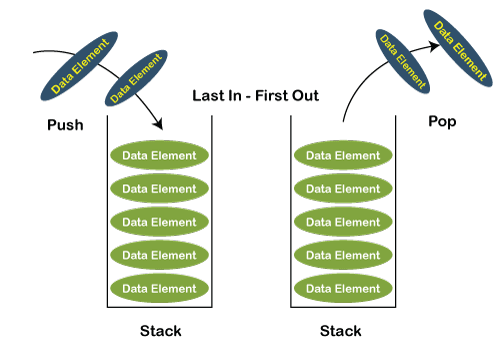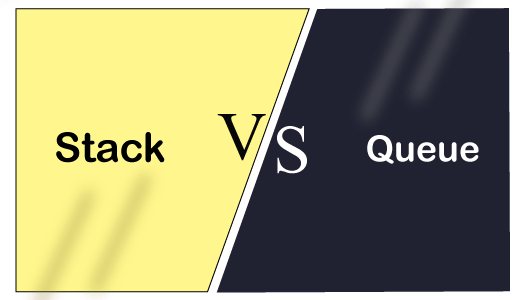# Stack vs. Queue

First, we will look at what is stack and what is queue individually, and then we will discuss the differences between stack and queue.

### What is a Stack?

A Stack is a linear data structure. In case of an array, random access is possible, i.e., any element of an array can be accessed at any time, whereas in a stack, the sequential access is only possible. It is a container that follows the insertion and deletion rule. It follows the principle LIFO (Last In First Out) in which the insertion and deletion take place from one side known as a top . In stack, we can insert the elements of a similar data type, i.e., the different data type elements cannot be inserted in the same stack. The two operations are performed in LIFO, i.e., push and pop operation.The following are the operations that can be performed on the stack:

• push(x): It is an operation in which the elements are inserted at the top of the stack. In the push function, we need to pass an element which we want to insert in a stack.
• pop(): It is an operation in which the elements are deleted from the top of the stack. In the pop() function, we do not have to pass any argument.
• peek()/top(): This function returns the value of the topmost element available in the stack. Like pop(), it returns the value of the topmost element but does not remove that element from the stack.
• isEmpty(): If the stack is empty, then this function will return a true value or else it will return a false value.
• isFull(): If the stack is full, then this function will return a true value or else it will return a false value.

In stack, the top is a pointer which is used to keep track of the last inserted element. To implement the stack, we should know the size of the stack. We need to allocate the memory to get the size of the stack. There are two ways to implement the stack:

• Static: The static implementation of the stack can be done with the help of arrays.
• Dynamic: The dynamic implementation of the stack can be done with the help of a linked list.

### What is the Queue?

A Queue is a linear data structure. It is an ordered list that follows the principle FIFO (First In -First Out). A Queue is a structure that follows some restrictions on insertion and deletion. In the case of Queue, insertion is performed from one end, and that end is known as a rear end. The deletion is performed from another end, and that end is known as a front end. In Queue, the technical words for insertion and deletion are enqueue() and dequeue(), respectively whereas, in the case of the stack, the technical words for insertion and deletion are push() and pop(), respectively. Its structure contains two pointers front pointer and rear pointer , where the front pointer is a pointer that points to the element that was first added in the queue and the rear pointer that points to the element inserted last in the queue.

### Similarities between stack and queue.

There are two similarities between the stack and queue:

• Linear data structure
Both the stack and queue are the linear data structure, which means that the elements are stored sequentially and accessed in a single run.
• Flexible in size
Both the stack and queue are flexible in size, which means they can grow and shrink according to the requirements at the run-time.

### Differences between stack and queue### The following are the differences between the stack and queue:

Basis for comparison Stack Queue
Principle It follows the principle LIFO (Last In- First Out), which implies that the element which is inserted last would be the first one to be deleted. It follows the principle FIFO (First In -First Out), which implies that the element which is added first would be the first element to be removed from the list.
Structure It has only one end from which both the insertion and deletion take place, and that end is known as a top. It has two ends, i.e., front and rear end. The front end is used for the deletion while the rear end is used for the insertion.
Number of pointers used It contains only one pointer known as a top pointer. The top pointer holds the address of the last inserted or the topmost element of the stack. It contains two pointers front and rear pointer. The front pointer holds the address of the first element, whereas the rear pointer holds the address of the last element in a queue.
Operations performed It performs two operations, push and pop. The push operation inserts the element in a list while the pop operation removes the element from the list. It performs mainly two operations, enqueue and dequeue. The enqueue operation performs the insertion of the elements in a queue while the dequeue operation performs the deletion of the elements from the queue.
Examination of the empty condition If top==-1, which means that the stack is empty. If front== -1 or front = rear+1, which means that the queue is empty.
Examination of full condition If top== max-1, this condition implies that the stack is full. If rear==max-1, this condition implies that the stack is full.
Variants It does not have any types. It is of three types like priority queue, circular queue and double ended queue.
Implementation It has a simpler implementation. It has a comparatively complex implementation than a stack.
Visualization A Stack is visualized as a vertical collection. A Queue is visualized as a horizontal collection.

Stack and queue both are a linear type of data structures both are used to store data in linear fashion

The basic difference between this two is

1. Stack stores data in LIFO manner it means last in first out. Last element push in the stack will be pop first. Push means insert an element into the stack and pop means delete an element from the stack. All the elements insert at the top of the stack and delete at top of the stack. We can implement stack with linked list.
2. Queue stores data in FIFO manner it means first in first out. For example line of students for registration first come student will be registere first. It means first enqueued element Will be dequeue first. Enqueue means insert an element in the queue and dequeue means delete an element from the queue. All the elements will be interest at rear and deleted from the front. We can implement queue with linked list.